Refer to our Texas Go Math Grade 2 Answer Key Pdf to score good marks in the exams. Test yourself by practicing the problems from Texas Go Math Grade 2 Unit 5 Assessment Answer Key.

Concepts and Skills

Question 1.
Use the tally chart to complete the pictograph. Draw a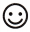for each bird. TEKS 2.10.B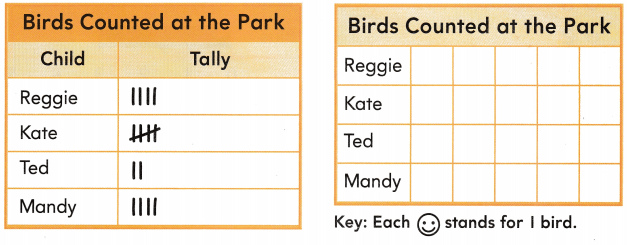Explanation:
Used the tally chart to complete the pictograph.
Drawn afor each bird.

Question 2.
Use the pictograph. How many birds did Kate count? TEKS 2.10.A
_____ birds
Explanation:
Kate count 5  birds in all

Question 3.
Use the data in the graph. Write a problem that you would use addition or subtraction to solve. Then solve. TEKS 2.10.C
Answer: Kate counted 5 and Ted counted 2. How many birds they counted altogether
5 + 2 = 7
Explanation:
Written a equation by using the addition.

Fill in the bubble for the correct answer choice.

Question 4.
The bar graph shows the marbles that Bev put into a bag. How many more blue marbles than green marbles are there? TEKS 2.10.A.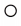324Explanation:
2 more blue marbles than green marbles are there.
3 – 1 = 2

Question 5.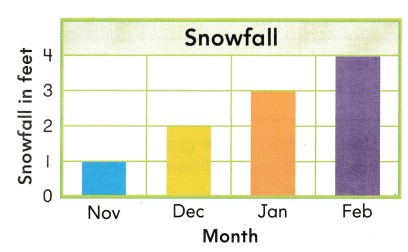The bar graph shows the snowfall in Sara’s town. Which describes the change that the data shows? TEKS 2.10.D.The amount of snow stayed the same.The amount of snow decreased.The amount of snow increased.
Answer: The amount of snow increased.
Explanation:
The change that the data shows is The amount of snow increased.

Texas Test Prep

Fill in the bubble for the correct answer choice.

Question 6.
Mr. Green made this pictograph. How many fewer books did Joy read than Tony? TEKS 2.10.A423
Explanation:
4 – 1 = 3 fewer books has Joy read than Tony

Question 7.
How many cards did Fran make? TEKS 2.10.B8106Explanation:
Fran make 3 x 2= 6 cards

Question 8.
Use the bar graph. How many mums are in the basket? TEKS 2.10.B846
Explanation:
There are 8 mums in the basket

Use this information to solve.

• There are 12 marbles in the jar.
• There are the same number of red marbles and blue marbles.Question 9.
Draw bars in the graph for the red marbles and blue marbles. TEKS 2.1o.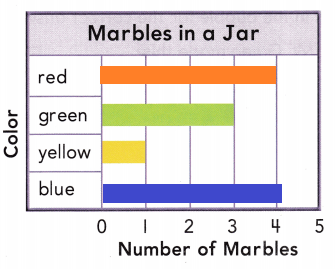Explanation:
There are 12 marbles in the jar.
There are the same number of red marbles and blue marbles.
Yellow and green combined is 4
12 – 4 = 8
as they are same each has 4

Question 10.
Explain how you decided how long the bars should be for red marbles and blue marbles. TEKS 2.10.A
Answer: Yellow and green combined is 4
12 – 4 = 8
as they are same each has 4
Explanation:
Yellow and green combined is 4
12 – 4 = 8
as they are same each has 4

Question 11.
Write three sentences to describe and compare the data in the bar graph. TEKS 2.10.A
Answer:  the 3 statements are stated below
Explanation:
Yellow and green combined is 4
There are the same number of red marbles and blue marbles.
red marbles and blue marbles is 8

Scroll to Top